Examples

Chapter 6 Class 8 Squares and Square Roots
Serial order wise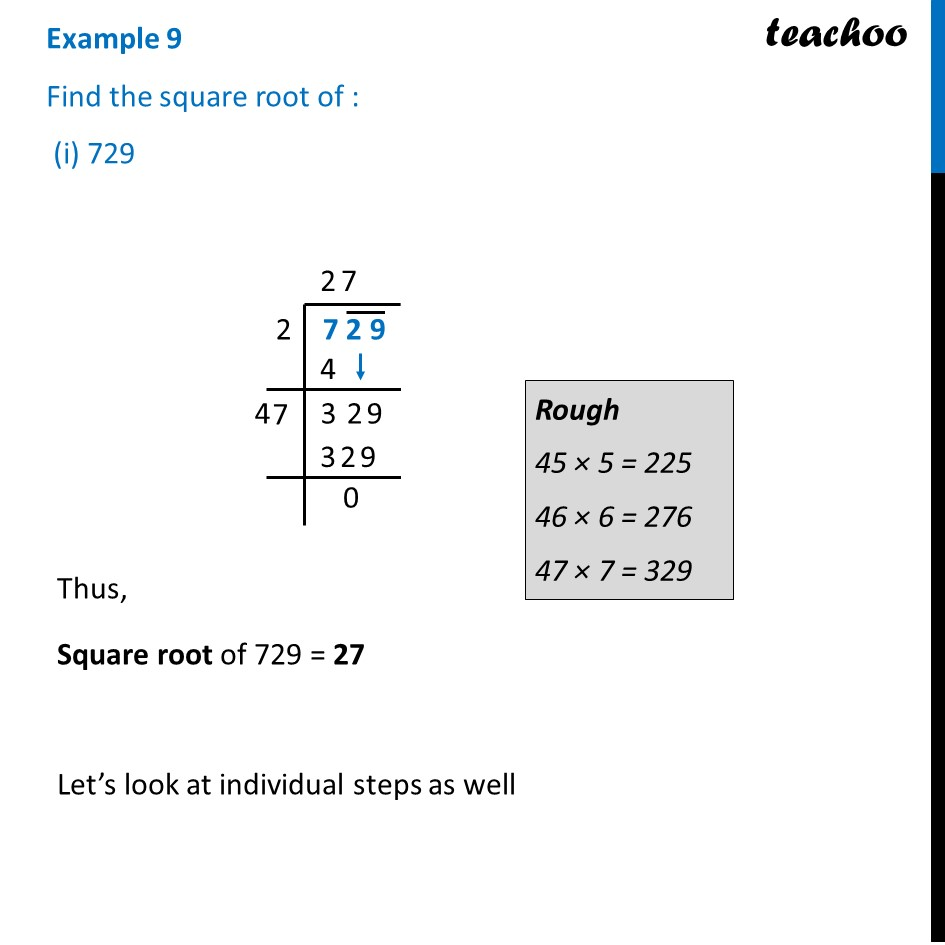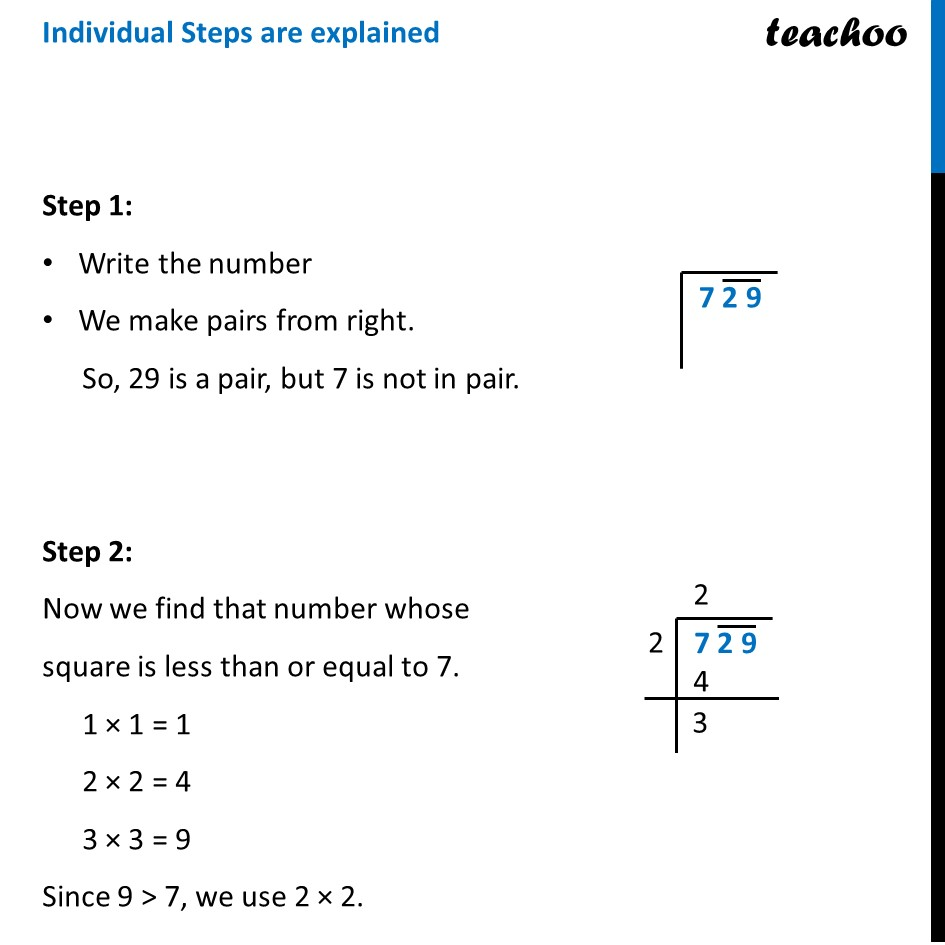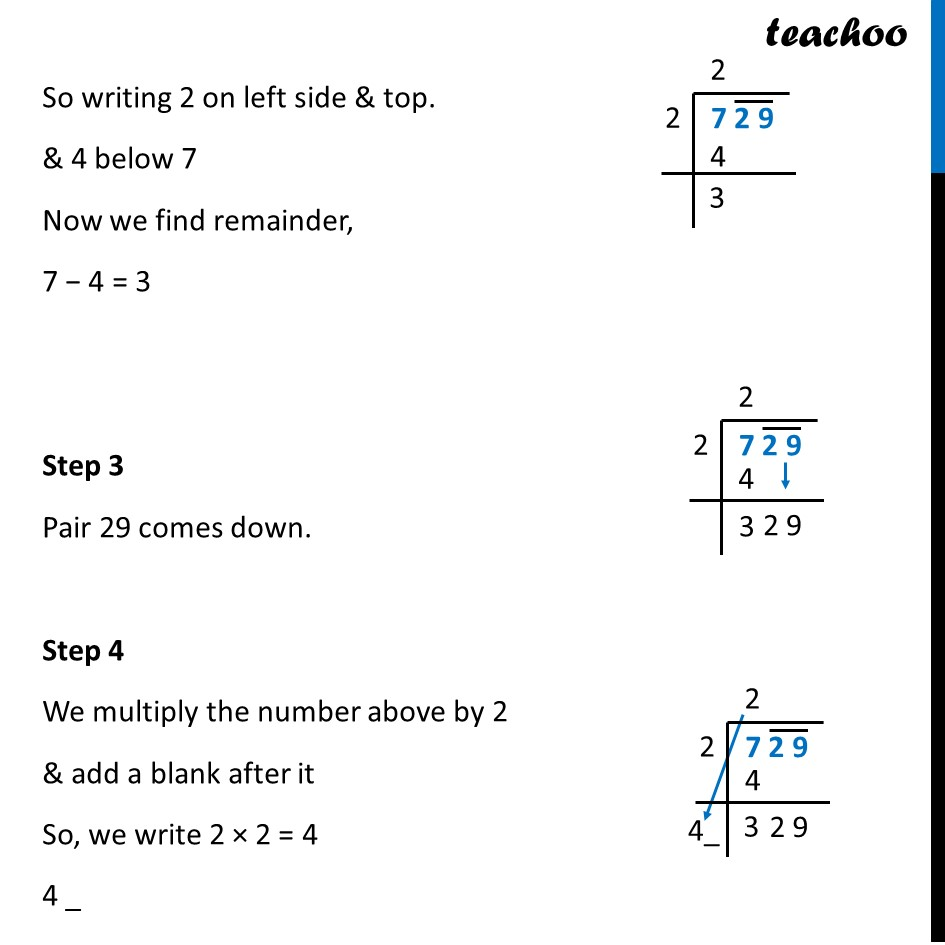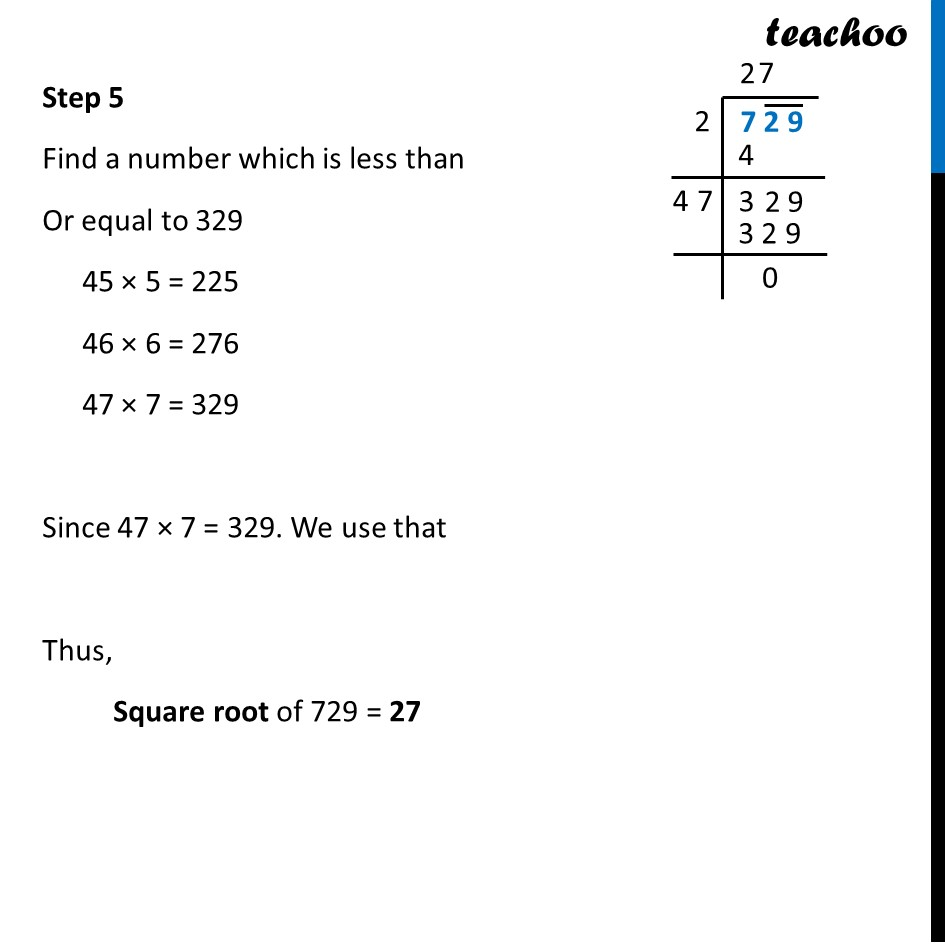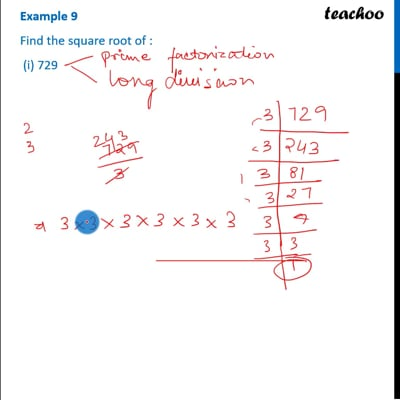This video is only available for Teachoo black users

Introducing your new favourite teacher - Teachoo Black, at only ₹83 per month

### Transcript

Example 9 Find the square root of : (i) 729 Rough 45 × 5 = 225 46 × 6 = 276 47 × 7 = 329 Thus, Square root of 729 = 27 Let’s look at individual steps as well Individual Steps are explained Step 1: Write the number We make pairs from right. So, 29 is a pair, but 7 is not in pair. Step 2: Now we find that number whose square is less than or equal to 7. 1 × 1 = 1 2 × 2 = 4 3 × 3 = 9 Since 9 > 7, we use 2 × 2. So writing 2 on left side & top. & 4 below 7 Now we find remainder, 7 − 4 = 3 Step 3 Pair 29 comes down. Step 4 We multiply the number above by 2 & add a blank after it So, we write 2 × 2 = 4 4 _ Step 5 Find a number which is less than Or equal to 329 45 × 5 = 225 46 × 6 = 276 47 × 7 = 329 Since 47 × 7 = 329. We use that Thus, Square root of 729 = 27